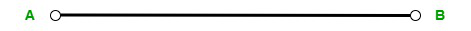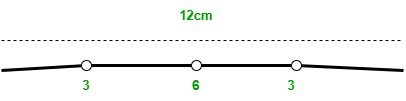Open In App

# How to divide a Line Segment into four equal parts?

Geometry is a part of mathematics that deals with the mathematical study of geometrical structures or figures which are plane shapes and solid shapes. It is also responsible for the calculation of the parameters of these shapes. The plane shapes of geometry include circle, square, rectangle, triangle, etc and the solid shapes include sphere, cube, cuboid, cone, prisms, pyramids, etc.

All the shapes involved in the study of geometry have their own distinct properties and derived formulas to calculate their parameters. These all shapes have vertices, faces, angles, edges, etc and all of them are formed by the joining of various points. It means all the shapes are formed by the relation between points, lines, and angles.

### What is a line?

The line is a one-dimensional and non-terminating geometrical structure. A line only consists of length, it does not have breadth or height as its dimensions.  A line does not have endpoints which means it extends endlessly in both directions. Hence, due to this endless extension, it is termed to be non-terminating. A line is composed of uncountable numbers of points and it is represented by any two points lying on it.Line

### What is a line segment?

A line segment is a portion of a line with two endpoints at both ends. As like a line, it is also a one-dimensional structure only with length. A line segment also moves in two opposite directions but is terminating in nature. Simply explaining, a line segment is actually the portion of a line lying between two endpoints. The segment terminates at these endpoints. Generally, a line segment is represented by a bar over the letters representing those two endpoints such as  \overline{\rm AB}.### How to divide a Line Segment into four equal parts?

The proportional sections of a line segment can be determined by calculating its midpoint. Suppose, let’s consider a line segment with endpoints A and B with a length of 12cm.Now, firstly we have two calculate the length of half of the line segment.

=>12/2

=>6cm

The length of half of the line segment will be 6cm

Then, calculate the half-length of the divided portion.

=>6/2

=>3cm

Hence, 1/4 of the line segment will be 3 cm.

Alternative method

We can directly divide the line segment into 4 equal proportions and calculate the length of each section.

=>12 x 1/4

=>12/4

=>3 cm

Hence, the length of 1/4 of the line segment is 3cm.

### Sample Problems

Problem 1. Find 1/2 of a line segment if its length is 14cm.

Solution:

The length of the given line segment is 14cm.

The 1/2 length of the line segment can be determined by

=>14 x 1/2

=>14/2

=>7cm

Hence, the 1/2 length of the line segment is 7cm.

Problem 2. Find the 1/4 of a line segment if its length is 32cm.

Solution:

The length of the given line segment is 32cm.

The 1/4 length of the line segment can be determined by

=>32 x 1/4

=>32/4

=>8cm

Hence, the 1/4 length of the line segment is 8cm.

Problem 3. Find the 1/4 of a line segment if its length is 48cm.

Solution:

The length of the given line segment is 48cm.

The 1/4 length of the line segment can be determined by

=>48 x 1/4

=>48/4

=>12cm

Hence, the 1/4 length of the line segment is 12cm.

Problem 4. Find the 1/6 of a line segment if its length is 36cm.

Solution:

The length of the given line segment is 36cm.

The 1/6 length of the line segment can be determined by

=>36 x 1/6

=>36/6

=>6cm

Hence, the 1/6 length of the line segment is 6cm.